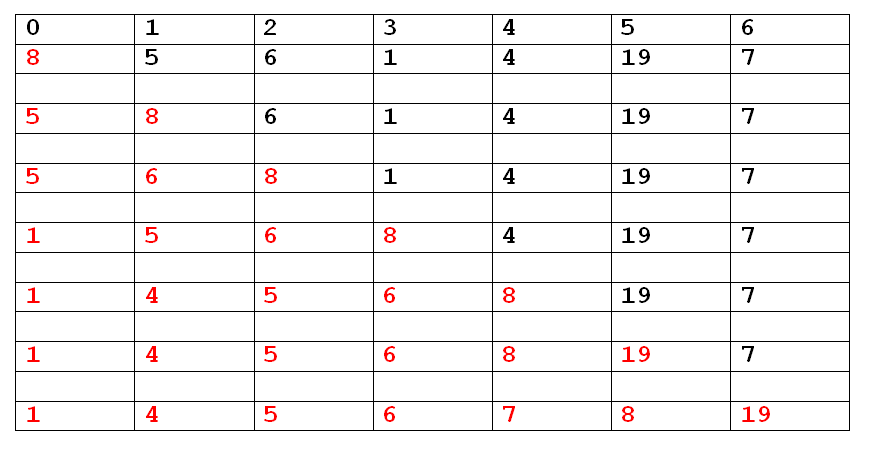# Insertion sort IN C, C++, Python, Java, Php

Insertion sort is based on the idea of inserting a record or records into an existing sorted file. To insert a record, we are required to find the proper place where the insertion is to be made, and then the record will be inserted.

The process will be as follows
Suppose we have an array A with “n” elements. A,A.. . . A[n-1].

Step 1: element A is considered it is alone so it is sorted.

Step 2: element A is considered. This element is either inserted before A or after A in such a manner that the elements A and A are sorted.

Step 3: element A is considered. This elements is either placed before A or between A and A or after A in such a manner that the elements A,A,A are sorted.
And so on.

Example:

Elements : 8,5,6,1,4,19,7After this step list automatically gets sorted (not required to check for the last element) so n-1 passes are required.

### C Implementation Of Insertion Sort    , C++ Implementation Of Insertion Sort    , Python Implementation Of Insertion Sort    , Java Implementation Of Insertion Sort    , PHP Implementation Of Insertion Sort

It is easy to learn
Few lines of code
Insertion sort is efficient for small data sets.
It does not change the relative order of elements with equal keys.

It is not effective for large number of sorting elements.
It needs a large number of element shifts
If we increase the number of elements then the performance of the program would be slow.

Complexity

In the insertion sort algorithm (n-1) times the loop will execute for comparisons and interchanging the numbers. The inner while loop iterates maximum of ((n-1)(*(n-1))/2 times to computing the sorting.

The number of comparisons in insertion can be easily computed.

Worst case: The worst case occurs when the elements in the array are in reverse order, then total number of comparisons are

(n-1) + (n-2) + . . . . + 3 + 2 + 1
n*(n-1)/2
which is Order of n2 i.e. O(n2)

Average case: Average number of comparisons can be found by dividing each term by 2. i.e. total number of comparisons are

(n-1)/2 + (n-2)/2 + . . . . + 3/2 + 2/2 + 1/2
n*(n-1)/2*1/2
n*(n-1)/4
which is Order of n2 i.e. O(n2)

Best case: Best case occurs when the elements of the array are already sorted, then only one comparison is made one each pass so the sort is of order n i.e. O(n).

C Insertion Sort

### C Implementation Of Insertion Sort

```/* insertion sort*/
#include<stdio.h>
#include<conio.h>
void main()
{
int a,i,j,t,n,min,pos,k;
clrscr();
printf("enter the total number of elements ");
scanf("%d",&n);
printf("enter the node elements \n");
for(i=0;i<=n-1;i++)
{
printf("enter the %d element ",i+1);
scanf("%d",&a[i]);
}
for(i=0;i<n;i++)
{
t=a[i];
j=i-1;
while((t<a[j]) && (j>=0))
{
a[j+1]=a[j];
j=j-1;
}
a[j+1]=t;
}
printf("sorted elements list is \n");
for(i=0;i<=n-1;i++)
{
printf("%d\n",a[i]);
}
getch();
}```

C++ Insertion Sort

### C++ Implementation Of Insertion Sort

```/* insertion sort*/
#include<iostream.h>
#include<conio.h>
void insertion(int [],int);

void main()
{
int a,i,n;
clrscr();
cout<<"enter the total number of elements ";
cin>>n;
cout<<"enter the elements "<<endl;
for(i=0;i<=n-1;i++)
{
cout<<"enter the element ";
cin>>a[i];
}
insertion(a,n);
cout<<"sorted elements list is \n";
for(i=0;i<=n-1;i++)
{
cout<<a[i]<<endl;
}
getch();
}

void insertion(int a[],int n)
{
int i,j,t;
for(i=0;i<=n-1;i++)
{
t=a[i];
j=i-1;
while((t<a[j]) && (j>=0)) /* to scan for the proper place*/
{
a[j+1]=a[j];
j=j-1;
}
a[j+1]=t;
}
}```

Python Insertion Sort

### Python Implementation Of Insertion Sort

```def insertion_sort(n):
for i in range(1, len(n)):
temp = n[i]
j = i - 1
while (j >= 0 and temp < n[j]):
n[j + 1] = n[j]
j = j - 1
n[j + 1] = temp
n = input('Enter the list of numbers: ').split()
n = [int(x) for x in n]
insertion_sort(n)
print('Sorted list: ', end='')
print(n)```

Output:

Enter the list of numbers: 5 2 3 6 9 8 7 4 2 1
Sorted list: [1, 2, 2, 3, 4, 5, 6, 7, 8, 9]
>>>

### Interview Questions

C Programming
C++ Programming
Class 11 (Python)
Class 12 (Python)
C Language
C++ Programming
Python

C Interview Questions
C++ Interview Questions
C Programs
C++ Programs

Important PagesNumber Of Visitors